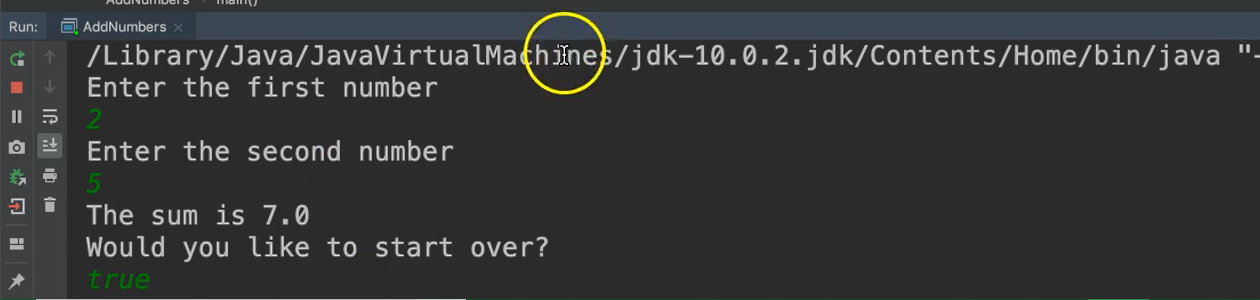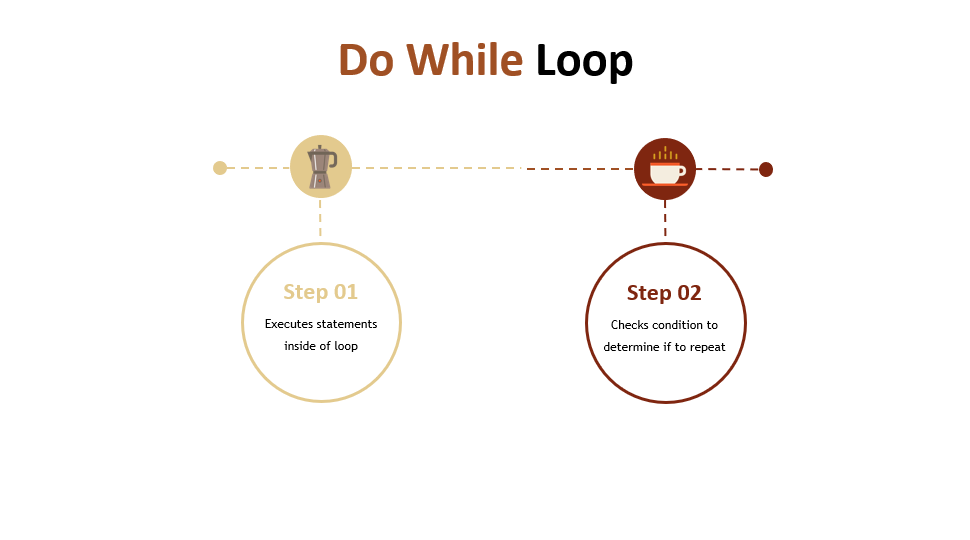Transcripted Summary

The programs that we wrote in the previous chapters did a calculation and then exited.

But what if you want to allow a user to run as many calculations as they like without having to restart the program each time?Let's write a program that allows a user to calculate the sum of two numbers as many times as they like to.

For this, we'll use the Do While Loop.

Okay, here we have a new class called `AddNumbers`.

Inside of the main method, what we want to do is:

• prompt the user to enter their first number
• then prompt them to enter the second number
• then ask them if they’d like to do another calculation

We're going to run this at least one time, and that's the purpose of the do while loop.

So, we'll write the word `do`, followed by the curly braces. Notice there's no condition here, because the do while loop doesn't check the condition until after the loop has been run one time.

So, we put all of the statements that we want to execute inside of the loop, then at the end of the loop, we add the `while` along with the condition. So, this is the format.

``````do{

} while(/*condition here*/);
``````

We'll go ahead and declare a sentinel variable called `again`. Let’s make `again` a boolean. And, we'll say use `again` as the condition for the `while`.

``````boolean again;

do{

} while(again);
``````

Because `again` is a boolean, I don't have to put any other operators in here. This is just going to be true or false anyway.

Let’s go inside of the loop, and we'll ask them for the first and second numbers then we'll sum them up.

``````boolean again;

do{

System.out.println("Enter the first number");
double num1 = scanner.nextDouble();

System.out.println("Enter the second number");
double num2 = scanner.nextDouble();

double sum = num1 + num2;

System.out.println("The sum is " + sum);

} while(again);
``````

Now we're going to need to update this sentinel because remember, the sentinel was set outside of the loop; but inside the loop, there has to be an opportunity for this to be updated.

So, we'll just go ahead and prompt the user and ask them if they want to run again.

``````System.out.println("Would you like to start over? True or False");
again = scanner.nextBoolean();
``````

``````package chapter4;

import java.util.Scanner;

/*
* DO WHILE LOOP:
* Write a program that allows a user to enter two numbers,
* and then sums up the two numbers. The user should be able to
* repeat this action until they indicate they are done.
*/

public static void main(String args[]){

Scanner scanner = new Scanner(System.in);

boolean again;

do{
System.out.println("Enter the first number");
double num1 = scanner.nextDouble();

System.out.println("Enter the second number");
double num2 = scanner.nextDouble();

double sum = num1 + num2;

System.out.println("The sum is " + sum);

System.out.println("Would you like to start over?");
again = scanner.nextBoolean();

} while(again);

scanner.close();
}
}
``````

Let's give it a go.

So, we'll say we enter 2 and 5.``````The sum is 7.0. Would you like to start over?
``````

Okay. So, it went inside of the loop, ran all of the input statements, and when it prompts us to start over, we actually need to enter “true” or “false”.

We'll do “true”, and then it'll say, "Enter the first number" again.

So, notice it goes back inside of the loop. This time let's say 2 and 3 and then this time we'll say “false”.

### # Key Points of Do While Loop• Just like the While Loop, it's also controlled by a condition.

• That condition is tested after the completion of the loop. So, the loop will always run at least once.

• So, it's good to use the do while loop when the loop should run at least one time, and based on the situation, they now need to repeat.

### # Steps of Do While Loop1. First the statements inside of the loop are executed. Again, be sure to update the sentinel here.
2. Then the condition is checked to determine if to run the loop again.

## Resources

Quiz

The quiz for Chapter 4 can be found at the end of Section 4d